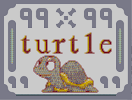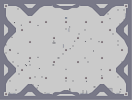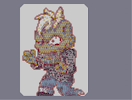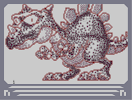### 99turtle99Hover over the thumbnail for a full-size version.

Author SilentDemon author:silentdemon bitesized n-art rated 2006-06-10 4 by 39 people. \$99turtle99#SilentDemon#none#11=00000000000000000:111900;1MQQQQQQQJ1<0;<061=000:1LOOOOOOOK1=0:110:000000000000000000000000;1<00000000000000;<000010100000000000000:11000:111100000000000000000000000000000000000000000;1<0000000000000000000010100000000000000000000:11110000000000000000000000000000000000000000000000000000000000000000;807<0000000000000000006=0:9000000000000000000001000000000000000000007<0;8000000000000000000:906=0000000000000000000000000000000000000000000000000000000000000000;1<0000000000000000000010100000000000000000000:111100000000000000000000000000000000000000000;1<00000000000000;<000010100000000000000:11000:111100000000000000000000000000000000000;<000<000;1MQQQQQQQJ1<0:110;1800:1LOOOOOOOK1=00007111<00000000000000000;11|12^156,216!12^156,222!12^150,228!12^144,234!12^138,240!12^132,240!12^144,240!12^150,240!12^156,240!12^156,234!12^150,234!12^156,228!12^162,240!12^168,240!12^174,240!12^144,246!12^144,252!12^144,264!12^144,258!12^144,270!12^144,276!12^144,282!12^144,288!12^156,246!12^156,258!12^156,252!12^150,252!12^150,246!12^150,258!12^150,264!12^156,270!12^156,264!12^150,270!12^150,276!12^156,276!12^156,282!12^150,282!12^150,288!12^156,288!12^156,294!12^156,300!12^162,306!12^168,312!12^174,306!12^180,300!12^180,306!12^174,312!12^168,318!12^144,294!12^144,300!12^144,306!12^150,312!12^156,318!12^156,312!12^162,312!12^162,318!12^156,306!12^150,306!12^150,300!12^150,294!12^168,306!12^174,300!12^180,294!12^444,216!12^444,222!12^444,228!12^438,228!12^432,234!12^438,234!12^444,234!12^450,240!12^456,240!12^462,240!12^444,240!12^438,240!12^432,240!12^426,240!12^420,240!12^432,246!12^432,252!12^432,258!12^432,264!12^432,270!12^432,276!12^432,288!12^432,282!12^432,294!12^432,300!12^432,306!12^438,312!12^444,318!12^450,318!12^456,318!12^462,312!12^468,306!12^468,300!12^462,306!12^456,306!12^450,306!12^444,306!12^438,306!12^444,312!12^450,312!12^456,312!12^468,294!12^462,300!12^444,246!12^438,246!12^438,258!12^438,252!12^444,252!12^444,258!12^444,300!12^444,294!12^444,288!12^444,282!12^444,276!12^444,270!12^444,264!12^438,300!12^438,294!12^438,288!12^438,282!12^438,276!12^438,270!12^438,264!12^534,318!12^546,318!12^540,318!12^540,312!12^534,312!12^534,306!12^534,300!12^540,306!12^546,306!12^546,312!12^546,300!12^540,300!12^528,318!12^534,294!12^534,288!12^534,282!12^534,276!12^534,270!12^534,264!12^534,258!12^534,252!12^534,240!12^534,246!12^534,234!12^534,228!12^534,222!12^534,216!12^540,288!12^540,294!12^546,294!12^546,288!12^546,282!12^540,282!12^540,276!12^540,270!12^540,264!12^540,258!12^540,252!12^540,246!12^540,240!12^540,234!12^540,228!12^540,222!12^540,216!12^546,276!12^546,270!12^546,264!12^546,258!12^546,252!12^546,246!12^546,240!12^546,234!12^546,228!12^546,222!12^546,216!12^528,216!12^522,216!12^516,216!12^528,210!12^534,210!12^534,204!12^540,204!12^546,198!12^546,204!12^546,210!12^540,210!12^522,210!12^516,318!12^522,318!12^528,312!12^552,312!12^552,318!12^558,318!12^564,318!12^228,240!12^228,246!12^228,252!12^228,258!12^228,264!12^228,270!12^228,276!12^228,282!12^228,288!12^228,294!12^228,300!12^234,306!12^240,312!12^246,318!12^258,318!12^252,318!12^264,318!12^270,312!12^276,306!12^282,300!12^282,294!12^282,288!12^282,282!12^282,276!12^282,270!12^282,264!12^282,258!12^282,252!12^282,246!12^282,240!12^276,240!12^270,240!12^264,240!12^270,246!12^276,246!12^276,252!12^276,258!12^276,270!12^276,264!12^276,276!12^276,282!12^276,288!12^276,294!12^276,300!12^270,306!12^264,312!12^258,312!12^252,312!12^246,312!12^240,306!12^234,300!12^234,294!12^234,288!12^234,282!12^234,276!12^234,270!12^234,264!12^234,258!12^234,252!12^234,246!12^234,240!12^222,240!12^222,246!12^216,240!12^240,300!12^246,306!12^282,306!12^276,312!12^282,312!12^282,318!12^288,318!12^288,312!12^300,306!12^294,312!12^336,240!12^330,240!12^324,246!12^318,252!12^324,252!12^330,246!12^336,246!12^342,252!12^342,258!12^342,264!12^342,270!12^342,276!12^342,282!12^342,288!12^342,294!12^342,300!12^342,306!12^336,312!12^330,318!12^324,318!12^336,318!12^342,318!12^348,318!12^354,318!12^360,318!12^366,318!12^372,318!12^360,312!12^354,312!12^348,312!12^342,312!12^354,306!12^348,306!12^348,300!12^354,300!12^354,294!12^348,294!12^348,288!12^354,288!12^354,282!12^348,282!12^354,276!12^348,276!12^348,270!12^354,270!12^354,264!12^348,264!12^348,258!12^354,258!12^354,252!12^348,252!12^348,246!12^342,246!12^342,240!12^348,240!12^354,240!12^354,246!12^360,252!12^366,246!12^366,252!12^360,258!12^372,246!12^372,240!12^378,240!12^378,234!12^384,240!12^372,252!12^378,252!12^384,246!12^378,246!12^384,234!12^390,240!12^384,252!12^390,246!12^630,318!12^636,318!12^642,318!12^648,318!12^624,318!12^654,318!12^660,312!12^666,306!12^666,300!12^666,294!12^618,312!12^612,306!12^612,300!12^612,294!12^606,288!12^606,282!12^606,276!12^606,270!12^612,264!12^612,258!12^612,252!12^618,246!12^624,240!12^630,240!12^636,240!12^642,240!12^648,240!12^654,240!12^660,246!12^666,252!12^666,258!12^666,264!12^660,306!12^654,312!12^648,312!12^660,300!12^642,312!12^636,312!12^630,312!12^624,312!12^624,306!12^618,306!12^618,300!12^618,294!12^612,288!12^612,282!12^612,276!12^612,270!12^618,264!12^618,258!12^618,252!12^624,246!12^630,246!12^624,252!12^636,246!12^642,246!12^648,246!12^654,246!12^660,252!12^660,258!12^660,264!12^654,264!12^648,264!12^642,264!12^636,264!12^630,264!12^624,264!12^654,252!12^654,306!12^606,294!12^606,264!12^396,552!12^390,552!12^384,552!12^378,552!12^372,552!12^366,552!12^360,552!12^354,552!12^348,552!12^342,552!12^336,552!12^330,552!12^318,552!12^324,552!12^312,552!12^306,552!12^300,552!12^294,552!12^288,552!12^282,552!12^276,552!12^270,552!12^264,552!12^258,552!12^402,552!12^408,552!12^414,552!12^420,552!12^426,552!12^432,552!12^438,552!12^444,552!12^450,552!12^456,552!12^462,552!12^468,552!12^474,552!12^480,552!12^486,552!12^492,552!12^498,552!12^504,552!12^510,552!12^516,552!12^522,552!12^528,552!12^534,552!12^540,552!12^546,552!12^552,552!12^564,552!12^558,552!12^570,552!12^258,540!12^264,540!12^276,528!12^276,522!12^276,516!12^276,510!12^282,522!12^288,516!12^294,516!12^282,504!12^282,498!12^288,492!12^300,510!12^306,510!12^312,510!12^306,504!12^306,498!12^294,486!12^282,474!12^324,516!12^324,528!12^324,534!12^324,540!12^324,546!12^318,546!12^330,546!12^336,546!12^342,546!12^330,522!12^336,528!12^342,528!12^348,528!12^354,528!12^288,468!12^282,468!12^270,468!12^276,468!12^264,462!12^258,462!12^252,462!12^246,456!12^240,456!12^234,450!12^306,444!12^228,444!12^222,444!12^216,438!12^210,432!12^204,426!12^204,420!12^204,414!12^204,408!12^210,402!12^210,396!12^216,402!12^222,402!12^216,384!12^216,390!12^222,378!12^222,372!12^228,366!12^234,360!12^240,354!12^246,348!12^252,348!12^252,354!12^252,366!12^246,372!12^246,378!12^228,396!12^234,390!12^240,384!12^240,366!12^234,366!12^234,372!12^234,378!12^234,384!12^240,378!12^240,372!0^246,360!12^252,360!12^258,342!12^264,342!12^270,336!12^276,336!12^288,336!12^282,336!12^294,336!12^300,336!12^306,336!12^312,336!12^318,336!12^324,336!12^312,342!12^330,342!12^336,342!12^342,348!12^318,348!12^324,354!12^324,360!12^306,342!12^300,342!12^294,342!12^288,348!12^282,354!12^282,360!12^276,366!12^276,372!12^276,402!12^276,396!12^276,390!12^276,384!12^276,378!12^282,414!12^282,408!12^288,420!12^294,426!12^300,426!12^306,426!12^312,426!12^318,420!12^324,414!3^245,363!12^324,372!12^318,366!12^312,366!12^306,366!12^300,366!12^294,366!12^288,366!12^282,372!12^282,378!12^282,384!12^282,390!12^288,384!12^288,378!12^288,372!12^360,456!12^360,450!12^360,444!12^360,438!12^360,432!12^360,426!12^360,408!12^360,402!12^360,396!12^360,390!12^360,384!12^360,378!12^360,372!12^366,462!12^366,468!12^366,474!12^372,474!12^378,492!12^390,516!12^396,522!12^402,528!12^390,528!12^396,528!12^384,534!12^378,534!12^372,534!12^366,534!12^360,534!0^295,357!0^305,351!0^314,357!3^314,361!3^305,361!3^312,351!12^414,540!12^408,546!12^378,480!12^384,492!12^390,498!12^402,510!12^408,510!12^414,510!12^420,510!12^426,516!12^432,516!12^438,516!12^444,516!12^450,516!12^456,516!12^462,516!12^468,522!12^474,522!12^480,528!12^486,528!12^498,534!12^504,534!12^510,534!12^510,528!12^516,540!12^516,546!12^522,546!12^528,546!12^516,522!12^522,516!12^528,516!12^534,510!12^540,510!12^546,510!12^552,516!12^570,528!12^576,534!12^582,540!12^588,546!12^582,552!12^576,552!12^558,510!12^558,504!12^558,498!12^558,492!12^558,486!12^552,480!12^546,474!12^540,468!12^534,468!12^528,468!12^522,462!12^522,474!12^516,480!12^510,486!12^504,492!12^498,498!12^486,504!12^474,504!12^480,504!12^468,504!12^462,504!12^456,504!12^438,498!12^432,492!12^426,492!12^420,486!12^414,480!12^414,474!12^408,468!12^402,456!12^402,450!12^396,444!12^384,438!12^378,432!12^366,432!12^372,432!12^372,402!12^378,396!12^384,390!12^390,384!12^396,378!12^402,378!12^408,372!12^414,366!12^420,366!12^426,366!12^432,366!12^438,366!12^444,366!12^450,366!12^456,372!12^462,372!12^468,378!12^474,384!12^486,396!12^498,420!12^498,414!0^282,516!0^282,510!0^288,510!0^294,510!0^294,504!0^288,504!0^288,498!0^294,498!0^294,492!0^300,498!0^300,504!0^366,456!0^366,450!0^366,444!0^372,450!0^378,444!0^384,444!0^372,438!0^390,444!0^378,450!0^378,456!0^372,456!0^372,462!0^372,468!0^378,468!0^378,462!0^384,462!0^384,456!0^390,456!0^396,450!0^396,456!0^390,462!0^390,468!0^384,468!0^396,468!0^402,468!0^402,462!0^402,474!0^396,474!0^390,474!0^384,474!0^378,474!0^384,480!0^390,480!0^396,480!0^402,480!0^408,480!0^390,486!0^402,486!0^396,486!0^414,486!0^420,492!0^414,492!0^408,492!0^402,492!0^396,492!0^390,492!0^396,498!0^402,504!0^402,498!0^414,498!0^408,498!0^408,504!0^414,504!0^420,504!0^420,498!0^426,498!0^426,504!0^426,510!0^432,510!0^432,504!0^432,498!0^438,504!0^438,510!0^444,510!0^444,504!0^450,504!0^450,510!0^456,510!0^462,510!0^468,516!0^468,510!0^474,510!0^474,516!0^480,522!0^480,516!0^480,510!0^486,510!0^486,516!0^486,522!0^492,528!0^492,522!0^492,522!0^492,516!0^492,510!0^492,504!0^498,504!0^498,510!0^498,516!0^498,522!0^498,528!0^504,528!0^504,522!0^504,516!0^504,510!0^504,504!0^504,498!0^510,522!0^510,516!0^510,510!0^510,504!0^510,498!0^516,516!0^516,510!0^516,504!0^516,498!0^516,492!0^516,486!0^522,510!0^528,510!0^528,504!0^522,504!0^522,498!0^522,492!0^522,480!0^534,474!0^540,480!0^552,510!0^552,504!0^546,504!0^540,504!0^534,504!0^528,498!0^528,492!0^528,486!0^534,486!0^534,492!0^534,498!0^540,498!0^540,492!0^540,486!0^546,486!0^552,486!0^552,492!0^552,498!0^546,498!0^546,492!0^330,528!0^330,534!0^330,540!0^336,540!0^336,534!0^342,534!0^354,534!0^354,546!0^348,546!0^348,540!0^342,540!0^354,540!0^360,540!0^366,540!0^372,540!0^378,540!0^384,540!0^390,540!0^396,540!0^396,534!0^408,540!0^402,540!0^402,546!0^396,546!0^390,546!0^384,546!0^378,546!0^372,546!0^366,546!0^360,546!3^550,505!3^543,502!3^534,504!3^528,509!3^509,518!3^501,526!3^491,523!3^477,518!3^466,516!3^444,513!3^434,513!3^419,505!3^407,505!3^390,493!3^385,478!3^371,467!3^381,463!3^511,510!11^804,588,510,474!11^804,588,510,468!11^804,588,510,462!11^804,594,504,480!11^804,588,498,486!11^804,588,492,492!11^810,594,480,498!11^804,594,468,498!11^804,594,456,498!12^450,498!11^810,588,480,492!11^810,588,486,486!11^810,594,492,480!11^798,582,498,474!11^810,594,498,468!11^810,582,498,462!11^798,600,510,456!11^798,588,498,456!11^798,582,504,450!11^810,600,486,474!11^798,606,480,480!11^852,486,474,486!11^828,558,468,492!11^840,528,486,468!11^840,504,486,462!11^822,546,486,456!11^804,564,492,450!11^846,546,504,444!11^852,558,504,438!12^510,438!12^510,444!12^516,456!12^516,450!11^858,528,456,492!11^822,552,444,492!11^816,582,432,486!11^834,570,444,486!11^858,510,456,486!11^846,528,468,486!11^846,534,468,480!11^840,516,474,474!11^792,534,426,480!11^828,546,438,480!11^822,534,450,480!11^852,522,462,480!11^906,516,462,474!11^834,546,450,474!11^804,546,438,474!11^810,522,426,474!11^828,522,474,468!11^828,504,474,462!11^798,516,420,468!11^810,504,462,468!11^822,480,432,468!11^846,480,444,468!11^864,492,456,468!11^876,528,462,462!11^882,510,474,456!11^894,528,450,462!11^870,552,438,462!11^822,594,426,462!11^846,594,414,462!12^408,462!11^858,570,462,456!11^882,540,492,444!11^888,498,480,444!11^888,486,480,450!11^864,474,468,450!11^900,492,492,438!11^900,474,498,432!11^882,468,498,426!12^504,432!12^504,426!11^828,486,450,456!11^846,474,438,450!11^876,498,438,456!11^864,510,450,450!11^822,498,456,450!11^858,546,426,456!11^822,510,414,456!11^816,522,414,450!11^882,516,426,450!11^816,510,468,444!11^816,510,444,444!11^846,522,432,444!11^870,546,456,444!11^870,438,480,438!11^870,468,468,438!11^846,450,456,438!11^870,450,444,438!11^894,450,432,438!11^828,462,420,444!11^876,468,408,444!11^888,540,396,438!11^876,504,408,438!11^810,528,420,438!12^390,438!11^834,504,486,432!11^810,510,474,432!11^906,552,462,432!11^846,504,450,432!11^852,546,438,432!11^888,558,426,432!11^876,576,414,432!11^864,600,402,432!11^864,588,390,432!11^882,522,486,426!11^816,486,474,426!11^816,474,462,426!11^840,498,450,426!11^858,510,438,426!11^858,492,426,426!11^840,480,414,426!11^864,522,402,426!11^888,492,390,426!11^822,528,378,426!11^894,564,372,426!11^846,546,486,420!11^828,546,486,414!11^828,504,474,420!11^864,462,462,420!11^840,570,450,420!11^846,468,438,420!11^828,450,426,420!11^798,492,414,420!11^798,474,402,420!11^804,456,390,420!11^864,498,378,420!11^816,492,366,420!12^360,420!11^852,486,474,414!11^804,474,462,414!11^822,504,450,414!11^852,528,438,414!11^810,492,426,414!11^864,516,486,408!11^888,522,474,408!11^822,522,414,414!11^822,504,402,414!11^876,534,390,414!11^870,522,378,414!11^846,510,366,414!12^366,408!12^360,414!11^894,582,462,408!3^368,456!3^429,514!11^846,493,450,408!11^870,486,438,408!11^876,576,426,408!11^822,612,414,408!11^846,612,402,408!11^876,600,390,408!11^900,600,378,408!11^870,612,384,402!11^828,576,390,396!11^888,594,396,390!11^846,576,474,402!11^834,534,462,402!11^846,528,450,402!11^852,540,438,402!11^834,606,426,402!11^846,606,414,402!11^858,600,402,402!11^882,600,396,402!11^792,564,402,396!11^816,564,414,396!11^846,564,426,396!11^870,558,438,396!11^792,546,450,396!11^822,534,462,396!11^792,534,474,396!11^792,516,408,390!11^786,498,420,390!11^840,516,432,390!11^846,546,444,390!11^906,552,456,390!11^882,516,468,390!11^810,534,402,384!11^822,504,414,384!11^816,522,426,384!11^822,534,438,384!11^858,540,450,384!11^828,468,462,384!11^876,540,456,378!11^828,528,444,372!11^858,534,444,378!11^816,522,414,378!11^822,504,432,378!11^840,528,420,372!11^900,522,426,378!11^858,468,432,372!0^516,468!0^504,468!0^492,468!0^480,468!0^468,468!0^456,468!0^444,468!0^432,468!0^420,468!0^486,480!0^474,480!0^462,480!0^450,480!0^438,480!0^426,480!0^444,492!0^456,492!0^468,492!0^480,492!0^492,492!0^492,444!0^480,444!0^468,444!0^456,444!0^504,444!0^444,444!0^432,444!0^420,444!0^408,444!0^498,480!0^510,480!0^510,456!0^498,456!0^486,456!0^474,456!0^462,456!0^450,456!0^438,456!0^426,456!0^414,456!0^402,432!0^414,432!0^426,432!0^438,432!0^390,432!0^450,432!0^462,432!0^474,432!0^486,432!0^498,432!0^456,420!0^468,420!0^480,420!0^492,420!0^444,420!0^432,420!0^420,420!0^408,420!0^396,420!0^384,420!0^372,420!0^378,408!0^390,408!0^402,408!0^414,408!0^426,408!0^438,408!0^450,408!0^462,408!0^474,408!0^486,408!0^468,396!0^480,396!0^456,396!0^444,396!0^432,396!0^420,396!0^408,396!0^396,396!0^384,396!0^402,384!0^414,384!0^426,384!0^438,384!0^450,384!0^462,384!0^444,372!0^432,372!0^420,372!12^486,402!12^492,408!12^480,390!12^522,468!12^444,498!12^492,498!3^509,471!3^498,484!3^485,493!3^473,499!3^455,498!3^446,492!3^431,487!3^461,490!3^473,490!3^485,484!3^494,477!3^498,473!3^507,462!3^461,494!3^499,494!3^460,480!3^475,480!3^489,470!3^506,453!3^493,454!3^475,455!3^458,474!3^436,477!3^426,470!3^370,427!3^370,416!3^370,412!3^379,424!3^392,429!3^404,438!3^407,447!3^414,453!3^416,467!3^425,474!3^448,482!3^460,463!3^476,468!3^447,470!3^434,462!3^426,452!3^446,458!3^426,463!3^456,452!3^469,452!3^482,445!3^493,443!3^501,442!3^493,431!3^482,429!3^477,433!3^469,437!3^474,443!3^459,442!3^450,442!3^442,449!3^426,445!3^427,433!3^445,429!3^455,427!3^475,416!3^499,418!3^471,400!3^449,412!3^414,414!3^401,411!3^389,402!3^457,390!3^438,396!3^418,403!3^451,377!3^400,387!3^435,412!11^852,426,576,546!11^876,426,564,546!11^840,432,552,546!11^894,408,540,546!12^534,546!11^900,396,570,540!11^858,390,558,540!11^864,390,546,540!11^864,414,534,540!11^834,438,528,540!11^894,432,522,534!11^888,402,534,534!11^840,408,546,534!11^882,384,558,534!11^894,378,564,534!11^822,426,522,528!11^798,438,534,528!11^816,438,546,528!11^798,426,558,528!12^564,528!11^828,414,528,522!11^810,408,540,522!11^834,396,552,522!12^558,522!11^798,408,540,516!11^834,390,504,546!11^870,414,492,546!11^804,510,480,546!11^888,606,468,546!11^900,618,456,546!11^882,618,444,540!11^864,630,444,546!11^906,636,432,546!11^888,636,426,546!11^876,624,426,540!11^882,618,438,540!11^846,600,456,540!11^834,576,468,540!11^822,582,480,540!11^804,546,492,540!11^828,546,480,534!11^864,552,468,534!11^828,558,456,534!11^828,576,444,534!11^834,546,438,534!11^846,546,426,534!11^840,564,414,534!11^870,534,468,528!11^780,528,456,528!11^774,534,444,528!11^780,552,432,528!11^798,552,420,522!11^846,534,420,528!11^876,528,432,522!11^828,522,444,522!11^774,522,456,522!11^852,540,414,528!11^858,528,408,522!11^804,552,414,516!11^840,528,402,516!11^822,540,390,510!11^822,522,390,504!11^822,504,384,498!11^870,498,378,486!12^384,504!12^396,504!3^400,499!12^384,486!0^384,498!0^390,510!0^408,522!0^420,522!0^432,522!0^444,522!0^456,522!0^450,534!0^438,534!0^426,534!0^414,534!0^462,534!0^474,534!0^486,534!0^420,546!0^432,546!0^444,546!0^456,546!0^468,546!0^480,546!0^492,546!0^504,546!12^492,534!0^522,534!0^528,522!0^540,522!0^552,522!0^534,534!0^546,534!0^558,534!0^540,546!0^552,546!0^564,546!0^576,546!0^570,534!11^834,408,312,516!11^786,474,306,516!11^906,516,312,522!11^840,522,300,522!11^906,522,294,522!11^888,528,312,528!11^906,510,300,528!11^846,522,288,528!11^906,534,312,534!11^840,534,312,540!11^882,540,306,546!11^828,552,294,546!11^876,546,300,540!11^822,552,300,534!11^786,528,288,540!11^810,534,288,534!11^834,552,276,534!11^822,534,276,540!11^792,540,264,546!11^810,534,282,546!11^768,558,276,546!0^318,522!0^306,522!0^294,522!0^300,534!0^312,534!0^306,546!0^294,546!0^282,546!0^270,546!0^258,546!0^288,534!0^276,534!12^270,534!12^252,546!12^324,522!12^414,546!12^408,534!12^384,510!12^378,498!3^522,531!3^531,538!3^547,543!3^406,521!3^426,520!3^443,524!3^455,523!3^463,528!3^433,524!3^414,520!3^477,532!3^491,537!3^414,532!3^426,538!3^435,542!3^427,547!3^421,546!3^317,517!3^311,522!3^299,522!3^288,525!3^281,533!3^315,543!3^306,548!3^317,537!3^317,531!3^297,550!3^288,549!3^274,538!3^304,516!3^426,546!3^540,548!3^284,516!3^285,509!3^289,496!3^333,542!3^343,547!3^357,549!3^371,550!3^384,550!3^399,550!3^407,544!11^816,390,378,528!11^798,390,366,528!11^864,372,384,522!11^846,378,372,522!11^882,366,360,522!11^900,366,348,522!11^816,372,342,522!11^840,366,336,516!11^858,360,348,516!11^798,372,360,516!11^822,354,372,516!11^840,348,378,516!11^870,348,372,510!11^894,348,360,510!11^852,336,348,510!11^870,330,336,510!11^894,330,321,343!11^852,378,324,510!12^318,510!11^804,354,372,504!11^852,630,366,492!11^870,528,366,498!11^858,498,366,504!11^828,534,354,504!11^822,546,354,498!11^810,546,354,492!11^774,576,342,504!11^780,588,342,498!11^780,606,330,504!11^804,624,318,504!11^828,630,318,498!11^786,624,330,498!11^810,636,342,492!11^828,342,330,492!11^810,342,318,492!11^840,330,306,492!12^300,492!11^822,330,366,486!12^372,486!11^798,336,354,486!11^804,312,342,486!11^822,312,330,486!11^846,312,318,480!11^870,312,318,486!11^894,312,306,486!11^804,288,294,480!12^288,480!11^834,288,306,480!11^816,288,330,480!11^840,432,342,480!11^864,324,354,480!11^876,324,366,480!12^372,480!11^858,300,354,474!11^876,294,342,474!11^858,282,330,474!11^894,294,318,474!11^882,276,306,474!11^900,276,294,474!11^798,264,354,468!11^822,258,342,468!11^822,276,330,468!11^840,270,318,468!11^864,258,306,468!11^840,252,300,468!11^888,258,276,462!11^894,252,288,462!11^798,246,300,462!11^816,240,312,462!11^798,228,324,462!11^906,234,336,462!11^906,450,348,462!11^906,426,258,456!11^900,438,270,456!11^906,462,282,456!11^888,462,294,456!11^876,468,306,456!11^870,558,324,456!11^888,480,318,456!11^876,474,336,456!11^858,474,348,456!11^810,498,354,462!11^870,480,312,450!11^906,468,324,450!11^888,504,342,450!11^828,474,336,450!11^852,456,348,450!11^810,450,336,444!11^834,456,324,444!11^900,474,318,444!11^888,504,294,444!11^846,474,282,444!11^798,450,270,444!11^876,438,258,444!11^888,390,504,540!3^504,540!3^486,456!3^498,462!3^480,468!3^468,468!11^864,438,246,444!11^876,432,240,444!12^240,450!12^246,450!12^252,450!12^258,450!12^264,450!12^270,450!12^276,450!12^288,450!12^282,450!12^294,450!12^300,450!11^906,474,348,444!11^888,450,228,438!11^834,456,240,438!11^840,444,252,438!11^852,264,264,438!11^852,234,276,438!11^876,246,288,438!11^888,234,300,438!11^828,222,312,438!11^840,240,324,438!11^888,384,336,438!11^870,228,348,438!11^894,342,348,432!11^798,390,336,432!11^858,480,324,432!11^882,510,312,432!11^864,510,300,432!11^834,558,288,432!12^306,444!11^894,480,276,432!11^900,438,264,432!11^864,444,258,432!11^822,456,246,432!11^870,480,234,432!11^840,504,222,432!11^906,522,216,426!11^900,498,348,426!11^810,216,336,426!11^846,216,324,426!11^834,204,330,420!11^870,210,342,420!11^888,216,348,420!11^852,204,228,426!11^798,204,240,426!11^810,186,252,426!11^816,204,264,426!11^900,216,276,426!11^876,192,282,426!11^792,186,336,414!11^834,192,348,414!11^858,186,336,408!11^900,192,348,408!11^900,198,336,402!11^900,192,348,402!11^846,282,336,396!11^882,210,348,396!11^828,342,336,390!11^906,312,348,390!11^846,330,336,384!11^870,318,348,384!11^852,366,336,378!11^810,378,348,378!11^852,384,348,372!11^828,378,336,372!12^330,408!12^330,402!12^330,396!12^330,390!12^330,384!12^330,378!12^330,372!11^846,438,336,366!12^330,366!11^852,384,348,366!11^810,396,348,360!12^348,354!12^354,366!12^354,360!11^900,396,336,360!11^864,432,336,354!11^870,378,330,348!11^864,402,282,342!11^828,420,276,342!11^876,516,276,348!11^876,432,264,348!11^864,432,264,354!11^864,414,270,354!11^846,444,270,360!11^900,438,264,360!11^846,432,264,366!11^804,438,258,372!11^792,414,270,372!11^792,414,258,378!11^816,420,264,378!11^834,420,252,384!11^810,390,264,384!11^852,462,264,390!11^864,426,264,396!11^804,450,264,402!11^846,468,252,390!11^906,414,252,396!11^840,432,252,402!11^834,408,246,390!11^906,456,240,396!11^828,444,240,402!11^900,474,234,402!11^894,516,249,408!11^791,452,249,415!11^858,486,270,408!11^804,408,270,414!11^906,462,264,408!11^816,480,264,414!12^258,408!12^258,414!11^864,468,276,420!11^822,438,264,420!11^888,420,252,420!11^876,378,240,420!11^876,420,228,420!11^906,432,216,420!11^906,426,234,414!11^804,408,234,408!12^240,408!12^240,414!11^888,444,222,414!11^846,420,216,414!11^870,474,216,408!11^798,456,222,408!0^372,516!0^360,516!0^348,516!0^336,516!0^384,516!0^348,492!0^360,492!0^372,492!0^336,492!0^324,492!0^312,492!0^366,528!0^378,528!0^354,504!0^330,504!0^318,504!0^342,504!0^366,504!0^378,504!0^354,480!0^342,480!0^330,480!0^366,480!0^318,480!0^306,480!0^294,480!0^300,468!0^312,468!0^324,468!0^336,468!0^348,468!0^360,468!0^306,456!0^318,456!0^330,456!0^342,456!0^354,456!0^294,456!0^282,456!0^270,456!0^258,456!0^312,444!0^324,444!0^336,444!0^348,444!0^300,444!0^288,444!0^276,444!0^264,444!0^252,444!0^240,444!0^246,432!0^258,432!0^270,432!0^282,432!0^294,432!0^306,432!0^318,432!0^330,432!0^342,432!0^354,432!0^234,432!0^222,432!0^228,420!0^240,420!0^252,420!0^216,420!0^264,420!0^276,420!0^324,420!0^336,420!0^348,420!0^354,408!0^342,408!0^348,396!0^336,396!0^342,384!0^354,384!0^348,372!0^336,372!0^342,360!0^336,348!0^270,408!0^234,408!0^222,408!0^210,408!0^252,408!0^264,396!0^252,396!0^240,396!0^246,384!0^258,384!0^252,372!0^264,372!0^258,360!0^270,360!0^264,348!0^276,348!0^288,342!3^408,426!3^384,414!3^382,521!3^366,526!3^371,505!3^347,514!3^329,504!3^352,493!3^352,472!3^336,487!3^314,485!3^328,468!3^345,449!3^321,452!3^307,464!3^302,474!3^384,525!3^376,516!3^351,525!3^332,512!3^296,471!3^290,476!3^308,486!3^336,429!3^353,400!3^277,458!0^150,216!0^150,222!0^144,228!0^138,234!0^126,240!0^150,318!0^144,312!0^168,300!0^174,294!0^138,306!0^138,300!0^138,294!0^138,288!0^138,282!0^138,276!0^138,270!0^138,264!0^138,258!0^138,258!0^138,246!0^138,252!0^210,240!0^216,246!0^222,252!0^222,258!0^222,264!0^222,270!0^222,276!0^222,282!0^222,294!0^222,300!0^222,288!0^228,306!0^234,312!0^240,318!0^276,318!0^294,306!0^264,306!0^270,300!0^270,294!0^270,288!0^270,282!0^270,276!0^270,270!0^270,264!0^270,258!0^270,252!0^264,246!0^258,240!0^312,252!0^318,246!0^324,240!0^360,246!0^366,240!0^372,234!0^318,318!0^330,312!0^336,306!0^336,300!0^336,294!0^336,288!0^336,282!0^336,276!0^336,270!0^336,264!0^336,258!0^336,252!0^438,318!0^432,312!0^456,300!0^462,294!0^426,306!0^426,300!0^426,294!0^426,288!0^426,282!0^426,276!0^426,270!0^426,264!0^426,258!0^426,252!0^426,246!0^414,240!0^426,234!0^432,228!0^438,222!0^438,216!0^510,216!0^516,210!0^528,204!0^540,198!0^510,318!0^522,312!0^528,306!0^528,300!0^528,294!0^528,288!0^528,282!0^528,276!0^528,270!0^528,264!0^528,258!0^528,246!0^528,246!0^528,240!0^528,234!0^528,228!0^528,222!0^528,252!0^654,258!0^648,252!0^618,240!0^612,246!0^606,258!0^606,252!0^600,294!0^600,288!0^600,282!0^600,276!0^600,270!0^600,264!0^660,294!0^654,300!0^648,306!0^618,318!0^612,312!0^606,306!0^606,300!4^108,570,1!4^120,570,1!4^132,570,1!4^96,570,1!4^144,570,1!4^156,570,1!4^168,570,1!4^180,570,1!4^192,570,1!4^204,570,1!4^216,570,1!4^228,570,1!4^240,570,1!4^264,570,1!4^252,570,1!4^276,570,1!4^288,570,1!4^300,570,1!4^312,570,1!4^324,570,1!4^336,570,1!4^360,570,1!4^348,570,1!4^372,570,1!4^384,570,1!4^396,570,1!4^420,570,1!4^408,570,1!4^432,570,1!4^444,570,1!4^456,570,1!4^468,570,1!4^480,570,1!4^492,570,1!4^504,570,1!4^516,570,1!4^528,570,1!4^540,570,1!4^552,570,1!4^564,570,1!4^576,570,1!4^588,570,1!4^600,570,1!4^612,570,1!4^624,570,1!4^636,570,1!4^648,570,1!4^660,570,1!4^672,570,1!4^684,570,1!4^696,570,1!0^330,360!0^318,342!12^312,342!12^318,336!12^294,342!12^288,336!12^240,450!12^252,450!12^264,450!12^276,450!12^288,450!12^300,450!12^306,444!8^732,180,2!8^732,192,2!8^732,204,2!8^732,216,2!8^732,228,2!8^732,240,2!8^732,252,2!8^732,264,2!8^732,276,2!8^732,288,2!8^732,300,2!8^732,312,2!8^732,324,2!8^732,336,2!8^732,348,2!8^732,360,2!8^732,372,2!8^708,180,0!8^708,192,0!8^708,204,0!8^708,216,0!8^708,228,0!8^708,240,0!8^708,252,0!8^708,264,0!8^708,276,0!8^708,288,0!8^708,300,0!8^708,312,0!8^708,324,0!8^708,336,0!8^708,348,0!8^708,360,0!8^708,372,0!8^84,180,2!8^84,192,2!8^84,204,2!8^84,216,2!8^84,228,2!8^84,240,2!8^84,252,2!8^84,264,2!8^84,276,2!8^84,288,2!8^84,300,2!8^84,312,2!8^84,324,2!8^84,336,2!8^84,348,2!8^84,360,2!8^84,372,2!8^60,180,0!8^60,192,0!8^60,204,0!8^60,216,0!8^60,228,0!8^60,240,0!8^60,252,0!8^60,264,0!8^60,276,0!8^60,288,0!8^60,300,0!8^60,312,0!8^60,324,0!8^60,336,0!8^60,348,0!8^60,360,0!8^60,372,0!0^72,180!0^72,192!0^72,204!0^72,216!0^72,240!0^72,228!0^72,252!0^72,264!0^72,276!0^72,300!0^72,288!0^72,312!0^72,324!0^72,336!0^72,348!0^72,360!0^72,372!0^720,180!0^720,192!0^720,204!0^720,216!0^720,228!0^720,240!0^720,252!0^720,264!0^720,276!0^720,288!0^720,300!0^720,312!0^720,324!0^720,336!0^720,348!0^720,360!0^720,372# -repost- request from nemetacyst

## Other maps by this authorCan't last forever Zombie Drake

Pages: (0)

Now I remember you more

### lol

hey, I'm doing well!

Yeah, actually - one of my new years resolutions was to make more maps.

5avesized.

FUCK DUDE

5/5

hes not...

yay!

### well kl

well good i loooooooooooooooooooooooooooooooooooooooooove tortoises!!! thats why my email is tortoisenator@googlemail.com

### Awesome!

That looks really cool!!

### Geeze dude, how much did that cost

And good art.
Even more Maplestory.

### bloody hell

how many exits did ya have to use to do that?
also nice art! and dont try to load this in Ned even on my Dell (3ghz pro 2gig ddr2 RAM with no other programs open)this took ages to load!